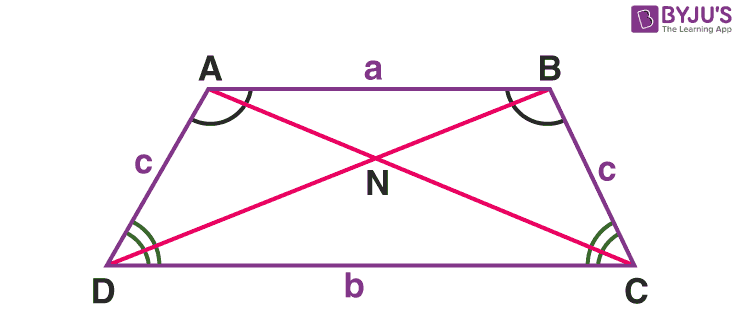# Properties of Isosceles Trapezium

A trapezium is a quadrilateral in which only one pair of opposite sides are parallel to each other.

An isosceles trapezium is a trapezium in which the non-parallel sides are equal in measure. In other words, the bases are parallel and the legs are equal in measure. In this article, we will learn the properties of an isosceles trapezium.

## What are the Properties of an Isosceles Trapezium?Consider the isosceles trapezium ABCD.

Property 1: Only one pair of sides are parallel. AB ∥ CD

Property 2: Non-parallel sides (legs) are equal in measure. AD = BC

Property 3: The diagonals are equal in measure. AC = BD

Property 4: The base angles are equal in measure. ∠D = ∠C

Property 5: The opposite angles are supplementary. ∠D +∠B = 1800 and ∠C +∠A = 1800

Property 6: The segment which joins the midpoints of the parallel sides is perpendicular to them.

Property 7: An isosceles trapezium can be inscribed in a circle.

Property 8: The diagonals form a pair of congruent triangles with equal sides as the base and the other pair – similar triangles.

### Area and Perimeter

Area of an isosceles trapezium

The area of an isosceles trapezium is given by the equation,

A = ½ h(a+b)

where, h is the perpendicular distance between the parallel sides, a and b are the lengths of the parallel sides.

Perimeter of the Isosceles Trapezium

The perimeter of the isosceles trapezium is given by the equation,

P = a + b + 2c

Where a and b are the lengths of the parallel sides and c is the common length of the legs AD and BC.